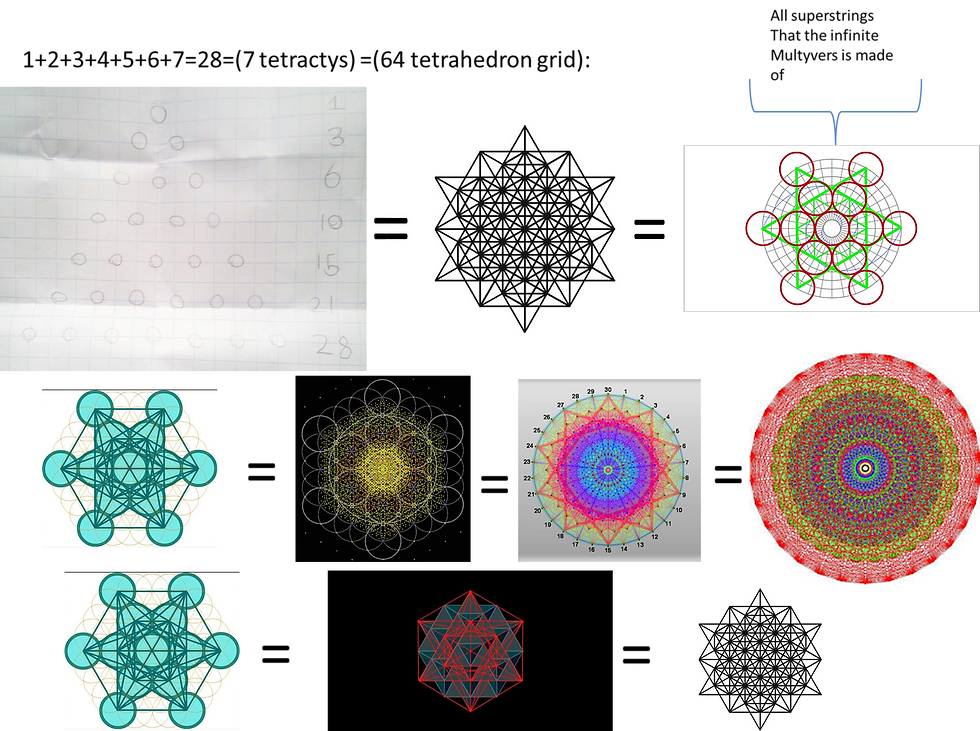top of page
Search

# 28 and 7 tetractys1+2+3+4+5+6+7=28=(7 tetractys)=(64 tetrahedron grid)=(Megatron's cube)=(fruit of life)

The 3D 7 tetractys is made out of 310 spheres and it encodes the infinite tetrahedron grid fractal which is the infinite dimensional E8 lie group fractal which is the infinite star dodecahedron fractal which is the fruit of life fractal which encodes all super strings

The 7 tetractys encodes the 7 colours which are 28